# NCERT Solutions for Class 7 Maths Chapter 9 Rational Numbers Ex 9.1

These NCERT Solutions for Class 7 Maths Chapter 9 Rational Numbers Ex 9.1 Questions and Answers are prepared by our highly skilled subject experts.

## NCERT Solutions for Class 7 Maths Chapter 9 Rational Numbers Exercise 9.1Question 1.
List five rational numbers between
(i) -1 and 0
(ii) -2 and -1
(iii) $$\frac{-4}{5}$$ and $$\frac{-2}{3}$$
(iv) $$\frac{-1}{2}$$ and $$\frac{2}{3}$$
(i) -1 and 0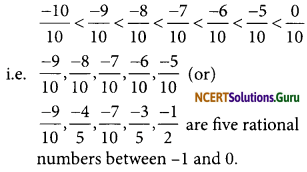(ii) -2 and -1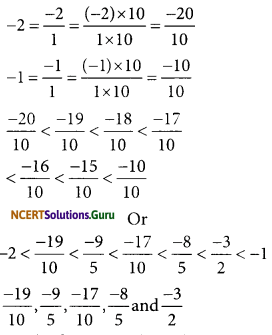are the five rational numbers between -2 and -1

(iii) $$\frac{-4}{5}$$ and $$\frac{-2}{3}$$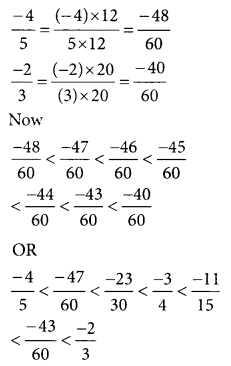The five rational numbers are
$$\frac{-47}{60}<\frac{-23}{30}<\frac{-3}{4}<\frac{-11}{15} \text { and } \frac{-43}{60}$$

(iv) $$\frac{-1}{2}$$ and $$\frac{2}{3}$$
L. C. M of 2 and 3 is 6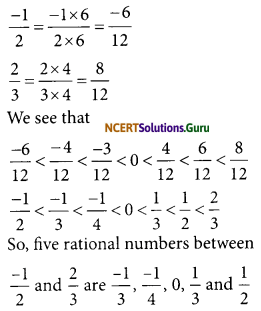Question 2.
Write four more rational numbers in each of the following patterns.
(i) $$\frac{-3}{5}, \frac{-6}{10}, \frac{-9}{15}, \frac{-12}{20}$$ ………………
(ii) $$\frac{-1}{4}, \frac{-2}{8}, \frac{-3}{12}$$ ………………
(iii) $$\frac{-1}{6}, \frac{2}{-12}, \frac{3}{-18}, \frac{4}{-24}$$ ………………
(iv) $$\frac{-2}{3}, \frac{2}{-3}, \frac{4}{-6}, \frac{6}{-9}$$ ………………Thus, we observe a pattern in these numbers.
The next four more rational numbers are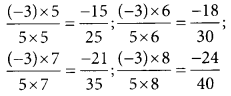The four required rational numbers are $$\frac{-15}{25}, \frac{-18}{30}, \frac{-21}{35}$$ and $$\frac{-24}{40}$$Thus, we observe a pattern in these numbers.
Next Four rational numbers are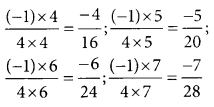The next four required rational numbers are $$\frac{-4}{16}, \frac{-5}{20}, \frac{-6}{24}$$ and $$\frac{-7}{28}$$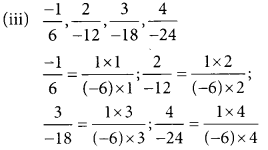Thus, we observe a pattern in these numbers
The next four rational numbers would beThe required four rational numbers are $$\frac{5}{-30}, \frac{6}{-36} ; \frac{7}{-42}$$ and $$\frac{8}{-48}$$Thus, we observe a pattern in these numbers
Four more rational numbers would beThe required four rational numbers are $$\frac{8}{-12}, \frac{10}{-15} ; \frac{12}{-18}$$ and $$\frac{14}{-21}$$

Question 3.
Give four rational numbers equivalent to:
(i) $$\frac{-2}{7}$$
(ii) $$\frac{5}{-3}$$
(iii) $$\frac{4}{9}$$
(i) Four rational numbers equivalent to(ii) Four rational numbers equivalent to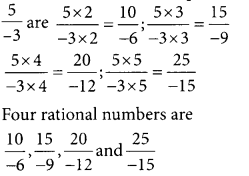(iii) Four rational numbers equivalent to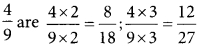Question 4.
Draw the number line and represent the following rational numbers on it:
(i) $$\frac{3}{4}$$
(ii) $$\frac{-5}{8}$$
(iii) $$\frac{-7}{4}$$
(iv) $$\frac{7}{8}$$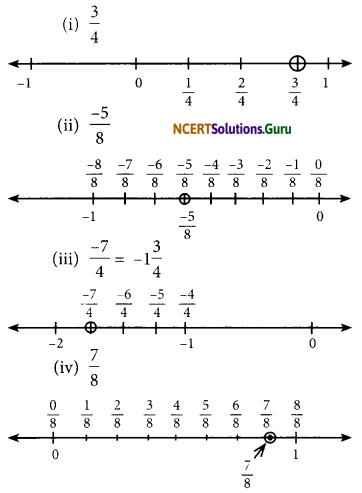Question 5.
ThepointsP,Q,RS,T,U,AandBonthe number line are such that, TR = RS = SU
and AP = PQ = QB. Name the rational numbers represented by P, Q, R, and S.Since AP = PQ = QB
Distance between 2 and 3 is divided into 3 equal parts.
Similarly, distance between -2 and -1 is also divided into three equal parts.
P represents the rational number
$$\left(2+\frac{1}{3}\right) \text { i.e. } \frac{7}{3}$$
Q represents the rational number
$$\left(2+\frac{2}{3}\right) \text { i.e. } \frac{8}{3}$$
R represents the rational number
$$\left(-1-\frac{1}{3}\right) \text { i.e. } \frac{-4}{3}$$
S represents the rational number
$$\left(-1-\frac{2}{3}\right) \text { ie } \frac{-5}{3}$$

Question 6.
Which of the following pairs represent the same rational number?
(i) $$\frac{-7}{21}$$ and $$\frac{3}{9}$$
(ii) $$\frac{-16}{20}$$ and $$\frac{20}{-25}$$
(iii) $$\frac{-2}{-3}$$ and $$\frac{2}{3}$$
(iv) $$\frac{-3}{5}$$ and $$\frac{-12}{20}$$
(v) $$\frac{8}{-5}$$ and $$\frac{-24}{15}$$
(vi) $$\frac{1}{3}$$ and $$\frac{-1}{9}$$
(vii) $$\frac{-5}{-9}$$ and $$\frac{5}{-9}$$
(i) $$\frac{-7}{21}$$ and $$\frac{3}{9}$$
Here $$\frac{-7}{21}$$ is a negative rational number and $$\frac{3}{9}$$ is a positive rational number.
$$\frac{-7}{21}=\frac{-1}{3} ; \frac{3}{9}=\frac{1}{3} ; \frac{-7}{21} \neq \frac{3}{9}$$
Thus, $$\frac{-7}{21}$$ and $$\frac{3}{9}$$ does not represent the same rational number.

(ii) $$\frac{-16}{20}$$ and $$\frac{20}{-25}$$
We have $$\frac{-16}{20}$$= $$\frac{-4}{5}$$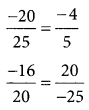Thus, $$\frac{-16}{20}$$ and $$\frac{20}{-25}$$ represent the same rational number.

(iii) $$\frac{-2}{-3}$$ and $$\frac{2}{3}$$
$$\frac{-2}{-3}=\frac{+2}{3} ; \frac{2}{3}=\frac{2}{3}$$
Thus, $$\frac{-2}{-3}$$ and $$\frac{2}{3}$$ represent the same rational number.

(iv) $$\frac{-3}{5}$$ and $$\frac{-12}{20}$$
We have, $$\frac{-3}{5}=\frac{-3}{5} ; \frac{-12}{20}=\frac{-3}{5}$$
So, $$\frac{-3}{5}=\frac{-12}{20}$$
Thus $$\frac{-3}{5}$$ and $$\frac{-12}{20}$$ represent the same rational number.

(v) $$\frac{8}{-5}$$ and $$\frac{-24}{15}$$
We have, $$\frac{8}{-5}=\frac{-8}{5} ; \frac{-24}{15}=\frac{-8}{5}$$
So, $$\frac{8}{-5}$$ = $$\frac{-24}{15}$$
Thus $$\frac{8}{-5}$$ and $$\frac{-24}{15}$$ represent the same rational number.

(vi) $$\frac{1}{3}$$ and $$\frac{-1}{9}$$
Here $$\frac{1}{3}$$ is positive integer and $$\frac{-1}{9}$$ is a negative integer.
∴ $$\frac{1}{3} \neq \frac{-1}{9}$$
Thus $$\frac{1}{3}$$ and $$\frac{-1}{9}$$ does not represent the same rational number.(vii) $$\frac{-5}{-9}$$ and $$\frac{5}{-9}$$
$$\frac{-5}{-9}$$ and $$\frac{5}{-9}$$ is a positive integer.
and $$\frac{-5}{-9}$$ = $$\frac{5}{-9}$$ is a negative integer
∴ $$\frac{-5}{-9} \neq \frac{5}{-9}$$
Thus $$\frac{-5}{-9}$$ and $$\frac{5}{-9}$$ do not represent tha same rational number.

Question 7.
Rewrite the following rational numbers in the simplest form:
(i) $$\frac{-8}{6}$$
(ii) $$\frac{25}{45}$$
(iii) $$\frac{-44}{72}$$
(iv) $$\frac{-8}{10}$$
(i) $$\frac{-8}{6}$$
$$\frac{-8}{6}=\frac{-4}{3}$$ (Divide both sides by 2)
The simplest form is $$\frac{-4}{3}$$

(ii) $$\frac{25}{45}$$
$$\frac{25}{45}=\frac{5}{9}$$ (Divide both sides by 5)
The simplest form is $$\frac{5}{9}$$

(iii) $$\frac{-44}{72}$$
$$\frac{-44}{72}=\frac{-11}{18}$$
Dividing both sides by 4)
The simplest $$\frac{-11}{18}$$

(iv) $$\frac{-8}{10}$$
$$\frac{-8}{10}=\frac{-4}{5}$$ (Dividing both sides by 2)
The simplest form is $$\frac{-4}{5}$$

Question 8.
Fill in the boxes with the correct symbol out of > , <, and =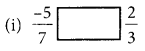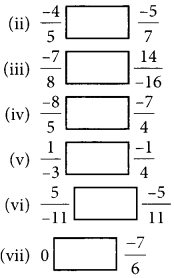$$\frac{-7}{6}$$ is a negative rational number. 6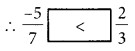(ii) $$\frac{-4}{5} \text { and } \frac{-5}{7}$$
are negative rational numbers.
L.C.M of 5 and 7 is 35.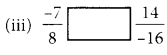are negative rational numbers.
L.C.M of 8 and 16 is 16.(iv) $$\frac{-8}{5}$$ and $$\frac{-7}{4}$$ are negative rational numbers.
LCM of 5 and 4 = 20(v) $$\frac{1}{-3}$$ and $$\frac{-1}{4}$$ negative rational numbers.
L.C.M of 3 and 4 is 12.(vi) $$\frac{5}{-11}$$ and $$\frac{-5}{11}$$ are negative rational numbers.(vii) $$\frac{-7}{6}$$ is a negative integer since 0 is 6
greater than every negative number.Question 9.
Which is greater in each of the following?
(i) $$\frac{2}{3}, \frac{5}{2}$$
(ii) $$\frac{-5}{6}, \frac{-4}{3}$$
(iii) $$\frac{-3}{4}, \frac{2}{-3}$$
(iv) $$\frac{-1}{4}, \frac{1}{4}$$
(v) $$-3 \frac{2}{7} ;-3 \frac{4}{5}$$
(i) $$\frac{2}{3}, \frac{5}{2}$$
L.C.M of 2 and 3 is 6Thus $$\frac{5}{2}$$ is greater rational number.

(ii) $$\frac{-5}{6}, \frac{-4}{3}$$
L.C.M of 6 and 3 is 6Thus $$\frac{-5}{6}$$ is greater rational number.

(iii) $$\frac{-3}{4}, \frac{2}{-3}$$
L.C.M of 4 and 3 is 12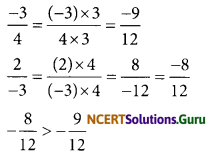$$\frac{2}{-3}>\frac{-3}{4}$$
Thus, the rational number $$\frac{2}{-3}$$ is greater.(iv) $$\frac{-1}{4}, \frac{1}{4}$$
since a positive rational number is always greater than a negative rational number.
$$\frac{1}{4}>\frac{-1}{4}$$
Thus, greater rational number is $$\frac{1}{4}$$

(v) $$-3 \frac{2}{7} ;-3 \frac{4}{5}$$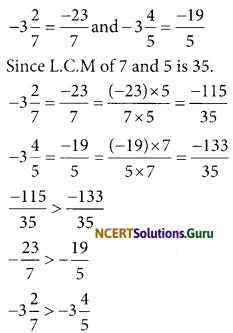Thus, rational number -3$$\frac{2}{7}$$ is greater.

Question 10.
Write the following rational numbers in ascending order.
(i) $$\frac{-3}{5}, \frac{-2}{5}, \frac{-1}{5}$$
(ii) $$\frac{-1}{3}, \frac{-2}{9}, \frac{-4}{3}$$
(iii) $$\frac{-3}{7}, \frac{-3}{2}, \frac{-3}{4}$$
(i) $$\frac{-3}{5}, \frac{-2}{5}, \frac{-1}{5}$$
$$-\frac{3}{5}<-\frac{2}{5}<-\frac{1}{5}$$
The ascending order is $$-\frac{3}{5},-\frac{2}{5},-\frac{1}{5}$$

(ii) $$\frac{-1}{3}, \frac{-2}{9}, \frac{-4}{3}$$
L.C.M of 3 and 9 is 9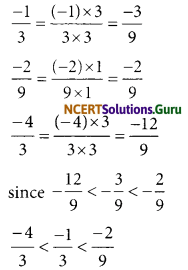Thus, the ascending order is
$$\frac{-4}{3}, \frac{-1}{3}, \frac{-2}{9}$$(iii) $$\frac{-3}{7}, \frac{-3}{2}, \frac{-3}{4}$$
L.C.M of 7, 2 and 4 is 28error: Content is protected !!